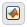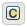## 使用 MATLAB 和 C 作为动作语言语法时的差异

•MATLAB® 作为动作语言。

•C 语言作为动作语言。

MATLAB 是新 Stateflow 图的默认动作语言语法。要创建使用 C 语言作为动作语言的图，请输入：

`sfnew -c`

### 比较动作语言的功能

C 构造：

• 递增与递减操作 `a++``a--`

• 赋值运算 `a += b``a –= b``a *= b``a /= b`

• 求值运算 `a != b``!a`

• 二元运算 `a %% b``a >> b``a << b``a & b``a | b`

• 注释标记 `//``/* */`

• MATLAB 函数

• 使用 MATLAB 作为动作语言的真值表

• 特殊赋值运算符 `:=`

• 上下文相关常量，例如 `4.3C`

• MATLAB 类型转换函数。例如 `single(x)`

• 具有类型关键字的 `cast` 函数。例如 `cast(x,"int8")`

• 具有 `"like"` 关键字的 `cast` 函数。例如 `cast(x,"like",z)`

• MATLAB 类型转换函数。例如 `uint16(x)`

• 具有 `type` 运算符的 `cast` 函数。例如 `cast(x,type(z))`

• 第一个索引

• 将最终值保存到基础工作区

• 单位

`Constant`, `Parameter`, `Input`, `Output``Local`, `Constant`, `Parameter`, `Input`, `Output`, `Temporary`

• 当您启用导入自定义代码时，状态和转移既支持自定义代码函数，也支持自定义代码变量（默认值）。

• 当您禁用导入自定义代码时，仅支持自定义代码函数。使用 `coder.ceval` (MATLAB Coder) 函数。

### 使用 MATLAB 作为动作语言的规范

#### 使用圆括号而不是方括号对向量和矩阵进行索引

`a(2,5) = 0;`

`a = 0;`

#### 使用 MATLAB 格式进行注释

`% This is a valid comment in the style of MATLAB`

C 样式注释，如 `//``/* */`，会自动更正为使用 %。

#### 用花括号将转移动作括起来

``E [x > 0] / {x = x+1;}``

``E [x > 0] / x = x+1;``